### Linear Regression Models

In matrix notation, a linear model is written as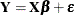whereis the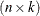design matrix (rows are observations and columns are the regressors),is the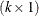vector of unknown parameters, andis the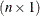vector of unobservable model errors. The first column ofis usually a vector of 1s and is used to estimate the intercept term.

The statistical theory of linear models is based on strict classical assumptions. Ideally, you measure the response after controlling all factors in an experimentally determined environment. If you cannot control the factors experimentally, some tests must be interpreted as being conditional on the observed values of the regressors.

Other assumptions are as follows:

• The form of the model is correct (all important explanatory variables have been included). This assumption is reflected mathematically in the assumption of a zero mean of the model errors,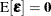.

• Regressor variables are measured without error.

• The expected value of the errors is 0.

• The variance of the error (and thus the dependent variable) for the ith observation is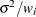, where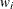is a known weight factor. Usually,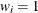for all i and thusis the common, constant variance.

• The errors are uncorrelated across observations.

When hypotheses are tested, or when confidence and prediction intervals are computed, an additional assumption is made that the errors are normally distributed.